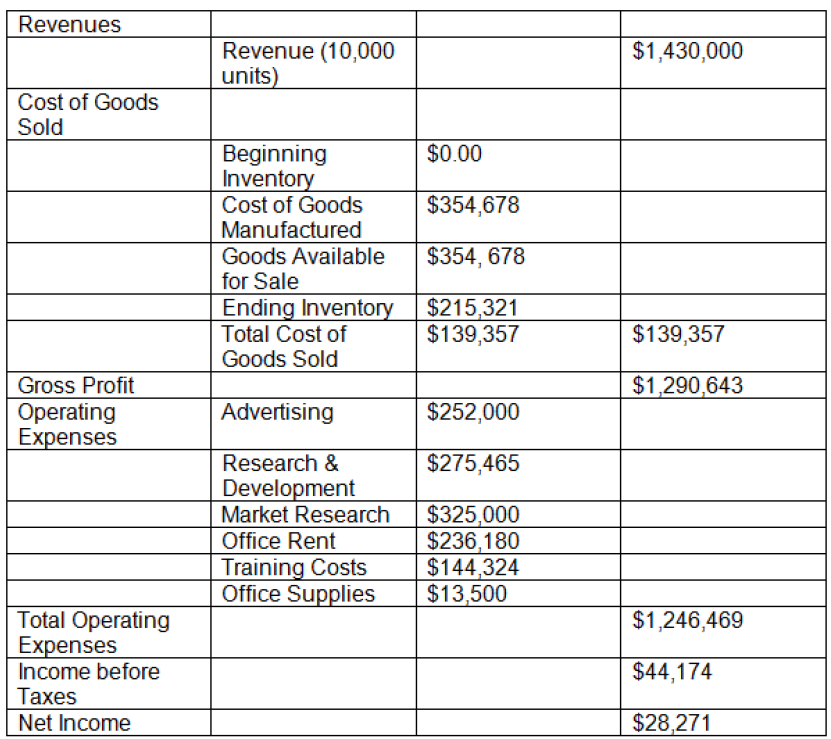# Financial Ratios

Source: Saylor

Student Price: FREE

This question pack looks at statement analysis and practical examples exploring problems with financial ratios.

Financial Ratios Question 1

A financial analyst examined the financial statements of a company from fiscal years: 2009, 2010, 2011, and 2012. This would be an example of which type of financial statement analysis?

A

Historical analysis

B

Vertical analysis

C

Horizontal analysis

D

Comparative analysis

Financial Ratios Question 2

Which of the following is NOT a ratio that can be calculated to determine a company's profitability?

A

Inventory turnover

B

Profit margin

C

Return on equity

D

Return on assets

Financial Ratios Question 3Review the following income statement for ABCD Builders. What can be concluded from the financial data?

A

The company’s operating expenses are higher due to the decrease in inventory held.

B

The company saw a net gain in profit due to the increase in inventory held.

C

The company discontinued operations that resulted in a higher cost of good sold.

D

The company is generating a low net income due to the high operating expenses.

Financial Ratios Question 4

Platinum Enterprises, Inc. had a net income in 2010 of \$1.2 million. In 2011, operations suffered after several plants were destroyed following an earthquake, and net income dropped to \$650,000. How would this affect the return on equity ratio for 2011? (Assume shareholders' equity remains constant.)

A

The return on equity would decrease by 35.5%.

B

The return on equity would decrease by 45.8%.

C

The return on equity would increase by 25.2%.

D

The return on equity would increase by 37.6%.

Financial Ratios Question 5

Historical financial statement ratios are often used to generate future financial statements when financial analysts conduct which of the following?

A

Pro‑forma estimation

B

Vertical analysis

C

Financial statement forecasting

D

Comparative analysis

Financial Ratios Question 6

In 2012, company AlphaBites generated a total revenue of \$125,000 with \$500,000 of total assets on the company's financial statements. The total overall expense for the fiscal year was recorded as \$88,500. What was the ROA for this firm?

A

4.5%

B

6.1%

C

7.3%

D

10.2%

Financial Ratios Question 7

Which of the equations is commonly used to determine a company's long‑term solvency?

A

Long‑Term Debt ÷ Current Assets

B

Total Debt ÷ Total Assets

C

Current Debt x Total Liabilities

D

(Equity + Total Debt) ÷ Total Assets

Financial Ratios Question 8

Toys‑A‑Bunch's 2012 financial statements were recently released to the public. Revenues totaled \$14.8 million, current assets totaled \$21.89 million, current liabilities totaled \$3.45 million, and long‑term liabilities remained unchanged at \$5.35 million. What is Toys‑A‑Bunch's working capital?

A

\$11.45 million

B

\$16.54 million

C

\$18.44 million

D

\$27.89 million

Financial Ratios Question 9

In 2012, Utility Queen recorded an EBIT (Earning before Income Tax) of \$535,000; \$1.35 million in shareholder's equity; an accounts payable balance of \$250,000; and \$385,000 in total liabilities. What was the company's debt-to‑equity ratio for 2011?

A

0.29

B

0.33

C

0.40

D

1.54

Financial Ratios Question 10

In 2012, company AlphaBites generated a total revenue of \$125,000 with \$500,000 of total assets on the company's financial statements. The total overall expense for the fiscal year was recorded as \$88,500. What was the asset turnover for this firm?

A

0.015

B

0.073

C

0.22

D

0.25

Financial Ratios Question 11

In 2011, Utility Queen recorded an EBIT (Earning before Income Tax) of \$505,000; accounts receivables balance of \$500,000; \$25,000 in interest expenses; and \$315,000 in long‑term debt. What was the company's interest coverage ratio for 2011?

A

0.05

B

12.6

C

14.6

D

20.2

Financial Ratios Question 12

Toys‑A‑Bunch's 2012 financial statements were recently released to the public. Revenues totaled \$14.8 million, current assets totaled \$21.89 million, current liabilities totaled \$3.45 million, and long‑term liabilities remained unchanged at \$5.35 million. Toys‑A‑Bunch's ______________ ratio was found to be 6.34.

A

quick

B

current

C

working

D

gross profit

CC BY 3.0 - Saylor - Llacey Simmons